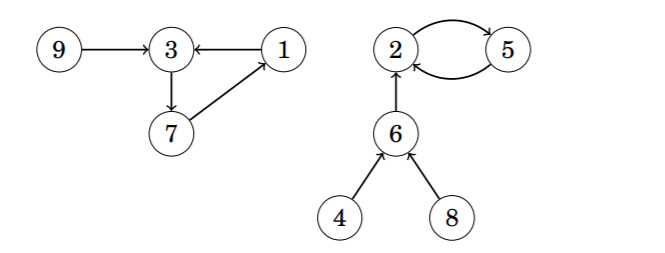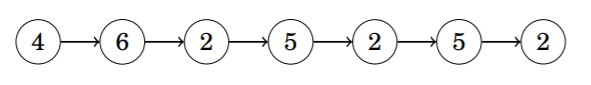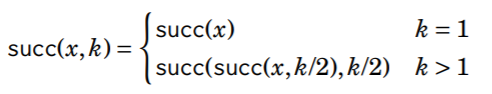Related Articles

# Successor Graph

• Last Updated : 28 Jan, 2021

A Successor Graph is a directed graph in which each vertex has outdegree one, i.e., exactly one edge starts at each node. A successor graph consists of one or more components, each of which contains one cycle and some paths that lead to it.

Successor graphs are sometimes called functional graphs. The reason for this is that any successor graph corresponds to a function that defines the edges of the graph. The parameter for the function is a node of the graph, and the function gives the successor of that node. For Example, the function

The above function defines the below graph:Since each node of a successor graph has a unique successor, a function succ(x, k) can also be defined that gives the node when a traversal begin at node x and walk k steps forward. For example, in the above graph succ(4, 6) = 2, because node 2 can be reached by walking 6 steps from node 4:A straightforward way to calculate a value of succ(x, k) is to start at node x and walk k steps forward, which takes O(k) time. However, using preprocessing, any value of succ(x, k) can be calculated in only O(logk) time.

The idea is to precalculate all values of succ(x, k) where k is a power of two and at most u, where u is the maximum number of steps we will ever walk. This can be efficiently done because we can use the following recursion:Precalculating the values takes O(n*log u) time because O(log u) values are calculated for each node. In the above graph, the first values are as follows:

After this, any value of succ(x, k) can be calculated by presenting the number of steps k as a sum of powers of two.
For example, if we want to calculate the value of succ(x, 11), we first form the representation 11 = 8 + 2 + 1. Using thatFor example, in the previous graphSuch a representation always consists of O(log k) parts, so calculating a value of succ(x, k) takes O(log k) time.

Attention reader! Don’t stop learning now. Get hold of all the important DSA concepts with the DSA Self Paced Course at a student-friendly price and become industry ready.  To complete your preparation from learning a language to DS Algo and many more,  please refer Complete Interview Preparation Course.

In case you wish to attend live classes with experts, please refer DSA Live Classes for Working Professionals and Competitive Programming Live for Students.

My Personal Notes arrow_drop_up